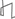Technical Reports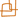Work Products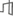Research Abstracts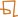Historical Collections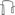Research Abstracts - 2006IntroductionLanguage, Learning, Vision & GraphicsPhysical, Biological & Social SystemsSystemsTheory### Bidimensionality and Algorithmic Graph Minors

#### Erik D. Demaine & MohammadTaghi Hajiaghayi

##### What

Downey and Fellows [DF99] introduced a new approach to cope with NP-hardness, called fixed-parameter tractability. For many NP-hard problems, the inherent combinatorial explosion can be attributed to a certain aspect of the problem, a parameter. The parameter is often an integer and small in practice. The running times of simple algorithms may be exponential in the parameter but polynomial in the rest of the problem size. For example, it has been shown that k-vertex cover (finding a vertex cover of size k) has an algorithm with running time O(k n + 1.271k) [CKJ01] and hence this problem is fixed-parameter tractable. A sample application of fixed parameter algorithms versus approximation algorithms is the installation of emergency service facilities such as fire stations. Here we suppose that we can afford to buy k fire stations to cover a city, and we require every building to be within r city blocks from the nearest fire station to ensure a reasonable response time. In this scenario, we can afford high running time (e.g., several weeks of real time) if the resulting solution builds fewer fire stations (which are extremely expensive) or has faster response time; thus, we prefer fixed-parameter algorithms over approximation algorithms.

In the above application, and many others, the graph is typically planar or nearly so. In fact, many problems which are intractable for general graphs allow polynomial-time solutions for structured classes of graphs, such as planar graphs and graphs of bounded ``treewidth''. The treewidth of a graph is a parameter measuring its similarity to trees, first introduced by Robertson and Seymour in their famous Graph Minor Theory [RS85]. Graphs of bounded treewidth inherit many good algorithmic properties of trees.

To obtain practical fixed-parameter algorithms on specific structured classes of graphs, we use the deep theory of Robertson and Seymour on graphs excluding a fixed graph H as a minor. Intuitively, the Robertson-Seymour theory says that, for any graph H, every H-minor-free graph can be expressed as a tree-structure of ``pieces'', where each piece is a graph which can be drawn in a surface in which H cannot be drawn, except for a bounded number of vertices called apex vertices and a bounded number of ``local areas of nonplanarity'' called vortices. Here the bounds depend only on H.

##### Progress

Because this graph-minor theorem is very general and has not appeared in print so far, not many other applications are known. In this project, we show many algorithmic consequences of this theorem and how this approach can be used as a guideline for solving other problems on H-minor-free graphs.

In one body of work [DHNRT06] we consider the class of graphs that minor-exclude a fixed graph that can be drawn in the plane with at most one crossing. Examples of such graphs include K3, 3-minor-free graphs and K5-minor-free graphs, and hence planar graphs are also a special case. We show that graphs in these classes can be efficiently decomposed into planar graphs and graphs of small treewidth; we use this decomposition to show that all such graphs have locally bounded treewidth (all local subgraphs are graphs of bounded treewidth). We make use of these structural properties to derive fixed-parameter algorithms and practical polynomial-time approximation schemes on this class of graphs for hereditary maximization problems such as maximum independent set, and for other problems such as maximum triangle matching, maximum H-matching, maximum tile salvage, minimum vertex cover, minimum dominating set, minimum edge-dominating set, and subgraph isomorphism for a fixed pattern.

We have since designed fixed-parameter and approximation algorithms for more general classes of graphs. In particular, in [DH04a] we study graphs that minor-exclude a fixed apex graph H, where in any apex graph there exists a vertex whose deletion produces a planar graph. Here we can obtain particularly good bounds on local treewidth, again using the Graph Minor Theory. On the other hand, we can generalize the ideas of fixed-parameter algorithms on planar graphs to graphs of bounded genus [DFHT06] and map graphs [DFHT05] which are both generalizations of planar graphs. Using the ideas of algorithms for apex-minor-free graphs and bounded-genus graphs, together with the seminal theorem of Robertson and Seymour on decomposition of any minor-excluding graph, we finally extend our suite of fixed-parameter techniques to general minor-excluding graphs [DFHT06].

Recently, in [DHK05], we developed a polynomial-time algorithm using topological graph theory to decompose a graph into the structure guaranteed by the Graph Minor Theory. This result has many applications. In particular, we show applications to developing many approximation algorithms, including a 2-approximation to graph coloring, constant-factor approximations to treewidth and the largest grid minor, combinatorial polylogarithmic-approximation to half-integral multicommodity flow, subexponential fixed-parameter algorithms, and PTASs for many minimization and maximization problems, on graphs excluding a fixed minor.

##### Future

See the survey [DH04b] for a more detailed description of this work and directions for future development.

##### References
[CKJ01]
Jianer Chen, Iyad A. Kanj, and Weijia Jia. Vertex cover: further observations and further improvements. Journal of Algorithms 41(2):280–301, 2001.
[DFHT05]
Erik D. Demaine, Fedor V. Fomin, MohammadTaghi Hajiaghayi, and Dimitrios M. Thilikos. Fixed-parameter algorithms for (kr)-center in planar graphs and map graphs. ACM Transactions on Algorithms, 1(1):33–47, July 2005.
[DFHT06]
Erik D. Demaine, Fedor V. Fomin, MohammadTaghi Hajiaghayi, and Dimitrios M. Thilikos. Subexponential parameterized algorithms on graphs of bounded-genus and H-minor-free graphs. Journal of the ACM, to appear.
[DH04a]
Erik D. Demaine and MohammadTaghi Hajiaghayi. Equivalence of local treewidth and linear local treewidth and its algorithmic applications. In Proceedings of the 15th Annual ACM-SIAM Symposium on Discrete Algorithms, pp. 833–842, New Orleans, Louisiana, January 2004.
[DH04b]
Erik D. Demaine and MohammadTaghi Hajiaghayi. Fast algorithms for hard graph problems: bidimensionality, minors, and local treewidth. In Proceedings of the 12th International Symposium on Graph Drawing, pp. 517–533, LNCS 3383, Harlem, New York, September 2004.
[DHK05]
Erik D. Demaine, MohammadTaghi Hajiaghayi, and Ken-ichi Kawarabayashi. Algorithmic graph minor theory: decomposition, approximation, and coloring. In Proceedings of the 46th Annual IEEE Symposium on Foundations of Computer Science, pp. 637–646, Pittsburgh, PA, October 2005.
[DHNRT06]
Erik D. Demaine, MohammadTaghi Hajiaghayi, Naomi Nishimura, Prabhakar Ragde, and Dimitrios M. Thilikos. Approximation algorithms for classes of graphs excluding single-crossing graphs as minors. Journal of Computer and System Sciences, 69(2):166–195, September 2004.
[DF99]
Rodney G. Downey and Michael R. Fellows. Parameterized Complexity. Springer-Verlag, New York, 1999.
[RS85]
Neil Robertson and Paul D. Seymour. Graph minors --- a survey. In I. Anderson, editor, Surveys in Combinatorics, pp. 153–171. Cambridge University Press, 1985.Computer Science and Artificial Intelligence Laboratory (CSAIL) The Stata Center, Building 32 - 32 Vassar Street - Cambridge, MA 02139 - USA tel:+1-617-253-0073 - publications@csail.mit.edu